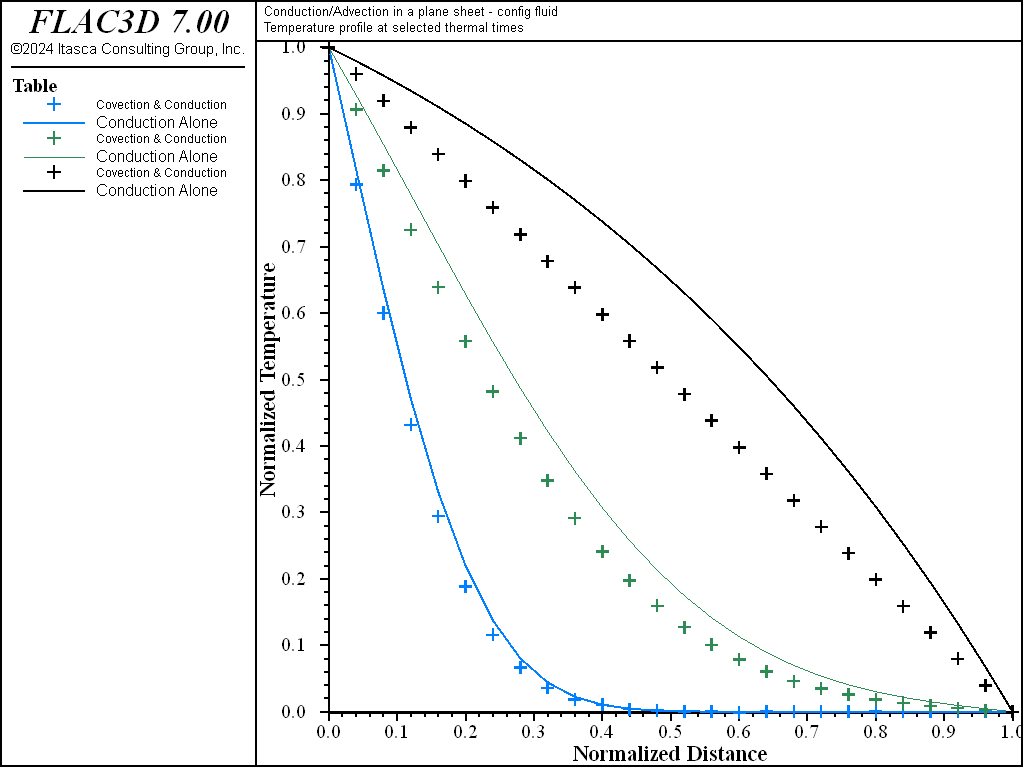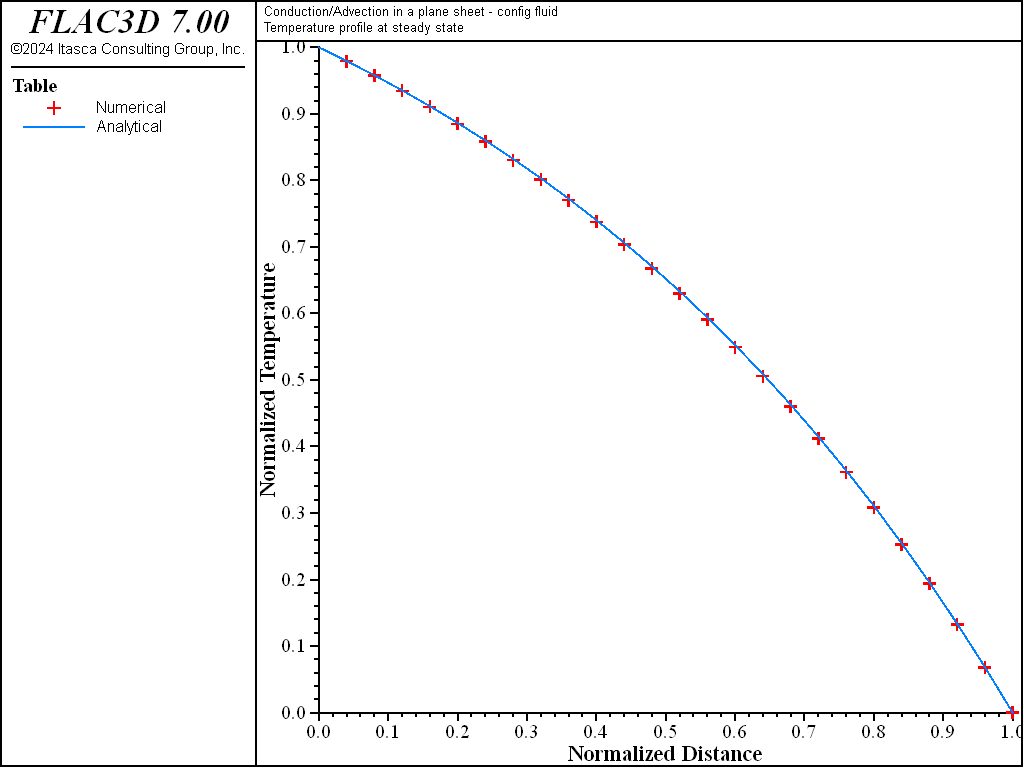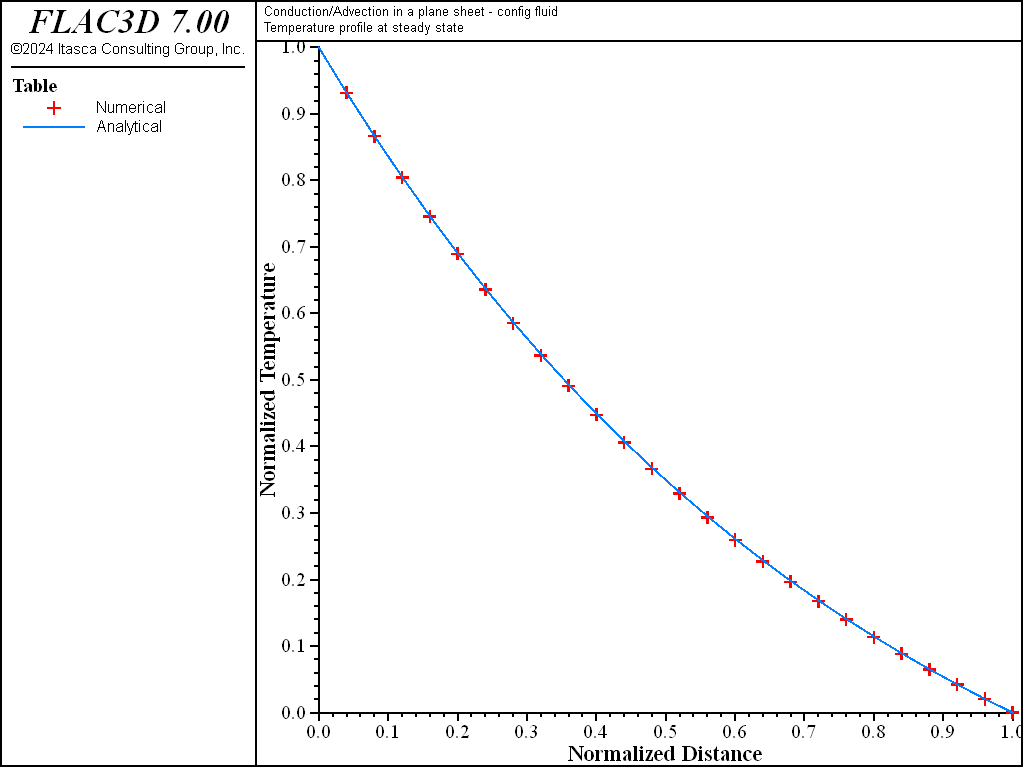# One-Dimensional Solution of Thermal Transport by Forced Convection and Conduction

Note

To view this project in FLAC3D, use the menu command Help ‣ Examples…. Choose “Thermal/ ConvectionAndConduction ThermalTransport” and select “ConvectionAndConduction ThermalTransport.prj” to load. The project’s main data files are shown at the end of this example.

This example illustrates the effect of forward and backward forced convection on the temperature profile, corresponding to forward conduction in a saturated plane sheet of thickness, $$L$$. Natural convection is not considered, and Darcy flux is constant throughout the simulation. The temperature is fixed at the top and bottom of the sheet. The temperature, initially uniform and equal to $$T_0$$, is raised suddenly to $$T_1$$ at the top. Its evolution is monitored in the sheet as time goes on.

At steady state, the one-dimensional form of the energy balance equation (this equation and the three that follow) may be expressed as

${{\partial^2 \hat T} \over {\partial \hat x^2}} - P_e {{\partial \hat T} \over {\partial \hat x}} = 0$

where $$\hat T = (T - T_0)/ T_0$$, $$\hat x = x / L$$, the origin of the coordinate is at the bottom of the sheet, $$x$$ is pointing up, $$P_e = \rho_0 c_w q_w L / k^T$$, $$P_e$$ is the Peclet number, and $$q_w$$ is the specific discharge in the $$x$$-direction.

The solution, with boundary conditions $$\hat T = 0$$ at $$\hat x = 0$$ and $$\hat T = \hat T_1$$ at $$\hat x = 1$$, is

$\hat T = \hat T_1 {{e^{P_e \hat x} - 1} \over {e^{P_e} - 1}}$

Global FISH constants and FISH functions to compare to the analytical solution are provided in the project. The Peclet number for the simulation has a magnitude of 1.54. The numerical solution for convection-conduction (solid line) is compared to the analytical solution for conduction (symbol) in Figure 1 and Figure 2. On these plots, the effect of fluid flow is seen to displace the temperature curves in the direction of the flow.

The FLAC3D temperature profile for convection-conduction is compared to the analytical solution at steady state in Figure 3 and Figure 4. As can be seen from these figures, the match is very good for this particular simulation.

The numerical simulation can be conducted with the fluid configuration (see “ConvectionAndConductionThermalTransportFluidOn.dat”) or without the fluid configuration (see “ConvectionAndConductionThermalTransportFluidOff.dat”), and with an attached grid (see “ConvectionAndConductionThermalTransportFluidAttach.dat”).

The stability of the numerical algorithm implemented in FLAC3D can be appreciated by exercising the files for various values of the grid Peclet and Courant numbers. For example, by varying grid density and specific discharge, the values of grid Peclet and Courant numbers for which the algorithm is stable may be derived. (See the discussion on numerical stability in Stability and Accuracy.)Figure 1: Comparison of temperature versus distance at three different times for convection and conduction acting in the same direction (solid lines) and conduction alone (symbols).Figure 2: Comparison of temperature versus distance at three different times for convection and conduction acting in opposite directions (solid lines) and conduction alone (symbols).Figure 3: Comparison of numerical (symbols) and analytical (solid lines) temperature versus distance profiles at steady state for convection and conduction acting in the same direction.Figure 4: Comparison of numerical (symbols) and analytical (solid lines) temperature versus distance profiles at steady state for convection and conduction acting in opposite directions.

Data Files

ConvectionAndConductionThermalTransportFluidOn.dat

model new
model title 'Conduction/Advection in a plane sheet - config fluid'
fish automatic-create off
model configure thermal fluid
;
zone create brick size 1 1 25 point 1 (0.1,0,0) point 2 (0,0.1,0) ...
point 3 (0,0,1)
zone face skin
; --- load fish constants and functions ---
program call 'fishfunctions' suppress
; --- thermal model ---
;  (no need to assign effective prop)
;  (mech model not needed to assign material density)
;  (f_thexp = 0, so there is no free convection)
zone thermal property conductivity 1.6 specific-heat 0.2
zone thermal property conductivity-fluid 0. specific-heat-fluid 0.2
; --- fluid model ---
zone fluid cmodel assign isotropic
zone fluid property permeability 2 porosity 0.5
zone fluid biot off
zone gridpoint initialize fluid-modulus 50
zone initialize fluid-density 1
zone initialize density 1000

zone face apply temperature 100 range group 'Bottom'
zone face apply temperature   0 range group 'Top'

; forward convection
zone face apply pore-pressure 5. range group 'Bottom'
zone face apply pore-pressure 0. range group 'Top'
model mechanical active off
model thermal active off
model fluid active on
model step 1000
model mechanical active off
model fluid active off
model thermal active on
model step 20
[cons]
[num_sol]
[ana_sol]
model step 80
[num_sol]
[ana_sol]
model step 900
[num_sol]
[ana_sol]
[ssac_sol]

; backward convection
zone face apply pore-pressure 5. range group 'Top'
zone face apply pore-pressure 0. range group 'Bottom'
model mechanical active off
model thermal active off
model fluid active on
model step 1000
model mechanical active off
model fluid active off
model thermal active on
model step 20
[cons]
[num_sol]
[ana_sol]
model step 80
[num_sol]
[ana_sol]
model step 900
[num_sol]
[ana_sol]
[ssac_sol]

program return


ConvectionAndConductionThermalTransportFluidOff.dat

model new
model title '  Conduction/advection in a plane sheet - not in config fluid'
fish automatic-create off
model configure thermal
;
zone create brick size 1 1 25 point 1 (0.1,0,0) point 2 (0,0.1,0) ...
point 3 (0,0,1)
zone face skin
; --- load fish constants and functions ---
program call 'fishfunctions' suppress
; --- thermal model ---
;     (f_thexp = 0 for no free convection)
zone thermal property specific-heat-fluid 0.2
zone thermal property conductivity-effective 1.6 specific-heat-effective 0.201
zone initialize density 1000
zone water density 1
zone face apply temperature 100 range group 'Bottom'
zone face apply temperature   0 range group 'Top'
model mechanical active off
model thermal active on

; forward convection
zone thermal property specific-discharge-x 0 specific-discharge-y 0 ...
specific-discharge-z 10.
model step 20
[cons]
[num_sol]
[ana_sol]
model step 80
[num_sol]
[ana_sol]
model step 900
[num_sol]
[ana_sol]
[ssac_sol]

; backward convection
zone thermal property specific-discharge-x 0 specific-discharge-y 0 ...
specific-discharge-z -10.
model step 20
[cons]
[num_sol]
[ana_sol]
model step 80
[num_sol]
[ana_sol]
model step 900
[num_sol]
[ana_sol]
[ssac_sol]

program return


ConvectionAndConductionThermalTransportFluidAttach.dat

model new
model title ...
'Conduction/Advection in a plane sheet - attached grid/config fluid'
fish automatic-create off
model configure thermal fluid
; --- load fish constants and functions ---
program call 'fishfunctions' suppress
; finer grid on top attached to a coarser grid below
zone create brick size 1 1 10 point 0 (0,0,0)     point 1 (0.1,0,0)   ...
point 2 (0,0.1,0)   point 3 (0,0,0.5)
zone create brick size 2 2 15 point 0 (0,0,0.5)   point 1 (0.1,0,0.5) ...
point 2 (0,0.1,0.5) point 3 (0,0,1)
zone attach by-face range position-z .499 .501
; --- thermal model ---
;  (no need to assign effective prop)
;  (mech model not needed to assign material density)
;  (f_thexp = 0, so there is no free convection)
zone thermal property conductivity 1.6 specific-heat 0.2
zone thermal property conductivity-fluid 0. specific-heat-fluid 0.2

zone fluid cmodel assign isotropic
zone fluid property permeability 2 porosity 0.5
zone fluid biot off
zone gridpoint initialize fluid-modulus 50

zone initialize density 1000
zone initialize fluid-density 1

zone face apply temperature 100 range position-z 0
zone face apply temperature   0 range position-z 1

; forward convection
zone face apply pore-pressure 5. range position-z 0
zone face apply pore-pressure 0. range position-z 1

; --- first establish flow field ---
model mechanical active off
model thermal active off
model fluid active on
model step 4000
; --- settings ---
model mechanical active off
model fluid active off
model thermal active on
; --- test ---
; (adv-cond plot is in solid line)
model step 20
[cons]
[num_sol]
[ana_sol]
model step 80
[num_sol]
[ana_sol]
model step 3600
[num_sol]
[ana_sol]
[ssac_sol]

; backward convection
zone face apply pore-pressure 5. range position-z 1
zone face apply pore-pressure 0. range position-z 0
; --- first establish flow field ---
model mechanical active off
model thermal active off
model fluid active on
model step 4000
; --- settings ---
model mechanical active off
model fluid active off
model thermal active on
; --- test ---
; (adv-cond plot is in solid line)
model step 20
[cons]
[num_sol]
[ana_sol]
model step 80
[num_sol]
[ana_sol]
model step 3600
[num_sol]
[ana_sol]
[ssac_sol]